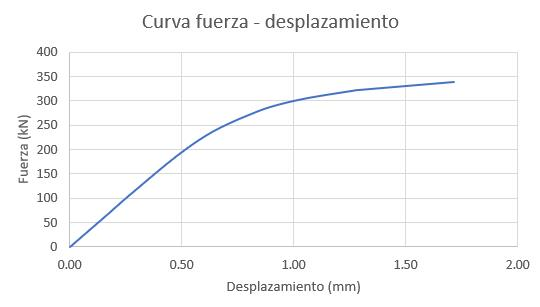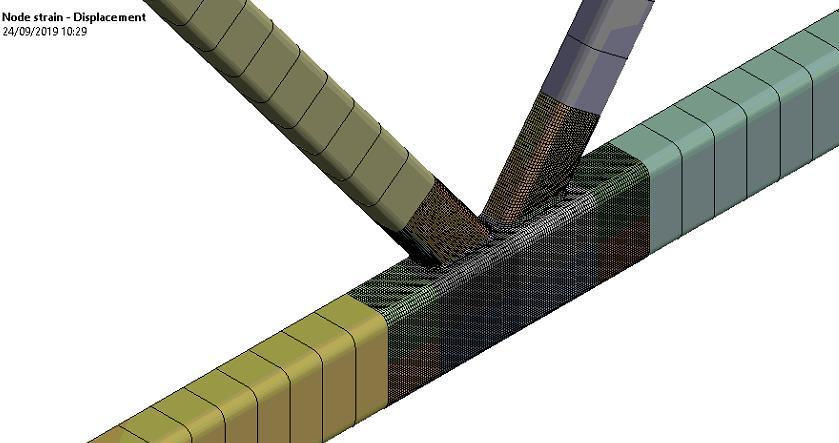## General Mechanical

•mekafime
Subscriber

Hello,

I have this curve which I get as a result of analyzing in Ansys, my question is, until when should I stop analyzing, I am increasing the strength and the curve continues to grow, but how do I define the fracture, under what criteria to stop increasing the force acting on the model ?.

Thanks•peteroznewman
Subscriber

This is the force-displacement curve which says nothing about fracture.

I assume you have defined a material using plasticity.

The material has a property called elongation.

Fracture occurs when the Total Equivalent Strain > Elongation.

Plot Total Equivalent Strain vs Displacement.

Increase the force until the Total Equivalent Strain > Elongation then you will have reached fracture.

•mekafime
Subscriber
•mekafime
Subscriber
•peteroznewman
Subscriber

What is the Elongation material property for your material?

I wonder if the top graph has mislabeled axes?  Isn't the vertical axis showing deformation in mm and the horizontal axis showing equiv. total strain?

•mekafime
Subscriber

Hi Peter,

A500 Gr.B

YS: 315 MPa

US: 400 MPa

23% Elongation

The graph was update.

•peteroznewman
Subscriber

Since Elongation is 0.23 and Total Strain > 0.23, that means the simulation has gone past the point where fracture would have occurred.

•mekafime
Subscriber

Hello Peter

In my curve I still do not reach 0.23 I am at 0.0035, I increase the strength and I am very far. To obtain the curve I am taking an analysis point (node) from which I also take the deformation, is the approach correct or should I take the strain of the entire model?•peteroznewman
Subscriber
It's the maximum total strain in the model that is compared to elongation.